# 26 Things I Learned in the Deep Learning Summer School

In the beginning of August I got the chance to attend the Deep Learning Summer School in Montreal. It consisted of 10 days of talks from some of the most well-known neural network researchers. During this time I learned a lot, way more than I could ever fit into a blog post. Instead of trying to pass on 60 hours worth of neural network knowledge, I have made a list of small interesting nuggets of information that I was able to summarise in a paragraph.

At the moment of writing, the summer school website is still online, along with all the presentation slides. All of the information and most of the illustrations come from these slides and are the work of their original authors. The talks in the summer school were filmed as well, hopefully they will also find their way to the web.

Alright, let’s get started.

## 1. The need for distributed representations

During his first talk, Yoshua Bengio said “This is my most important slide”. You can see that slide below: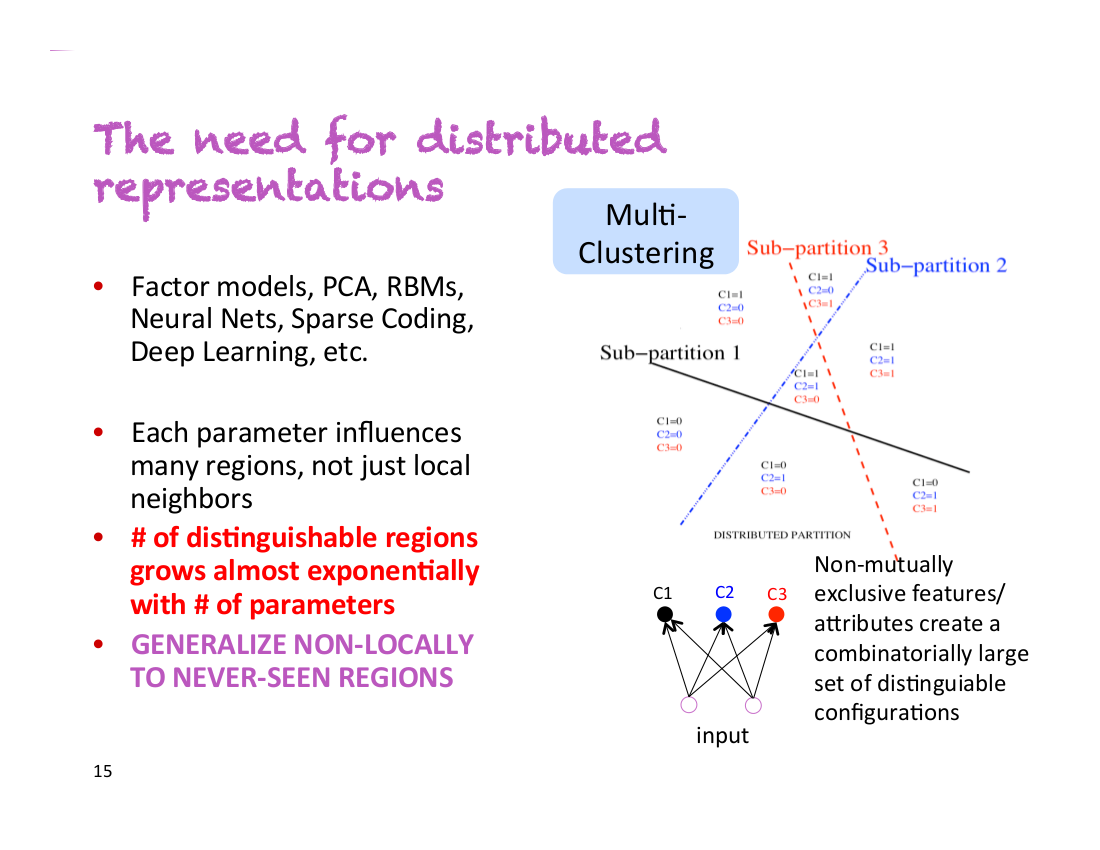Let’s say you have a classifier that needs to detect people that are male/female, have glasses or don’t have glasses, and are tall/short. With non-distributed representations, you are dealing with 2*2*2=8 different classes of people. In order to train an accurate classifier, you need to have enough training data for each of these 8 classes. However, with distributed representations, each of these properties could be captured by a different dimension. This means that even if your classifier has never encountered tall men with glasses, it would be able to detect them, because it has learned to detect gender, glasses and height independently from all the other examples.

## 2. Local minima are not a problem in high dimensions

The team of Yoshua Bengio have experimentally found that when optimising the parameters of high-dimensional neural nets, there effectively are no local minima. Instead, there are saddle points which are local minima in some dimensions but not all. This means that training can slow down quite a lot in these points, until the network figures out how to escape, but as long as we’re willing to wait long enough then it will find a way.

Below is a graph demonstrating a network during training, oscillating between two states: approaching a saddle point and then escaping it.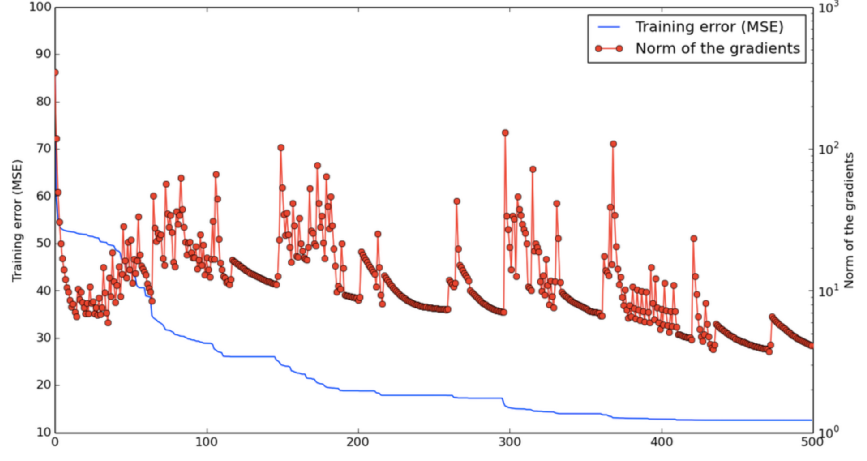Given one specific dimension, there is some small probability $$p$$ with which a point is a local minimum, but not a global minimum, in that dimension. Now, the probability of a point in a 1000-dimensional space being an incorrect local minimum in all of these would be $$p^{1000}$$, which is just astronomically small. However, the probability of it being a local minimum in some of these dimensions is actually quite high. And when we get these minima in many dimensions at once, then training can appear to be stuck until it finds the right direction.

In addition, this probability $$p$$ will increase as the loss function gets closer to the global minimum.  This means that if we do ever end up at a genuine local minimum, then for all intents and purposes it will be close enough to the global minimum that it will not matter.

## 3. Derivatives derivatives derivatives

Leon Bottou had some useful tables with activation functions, loss functions, and their corresponding derivatives. I’ll keep these here for later.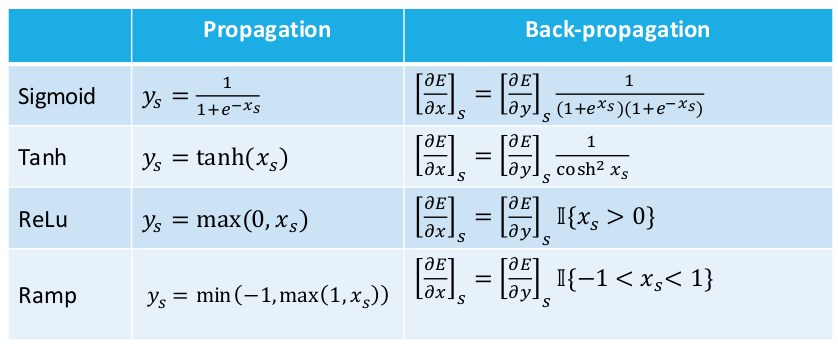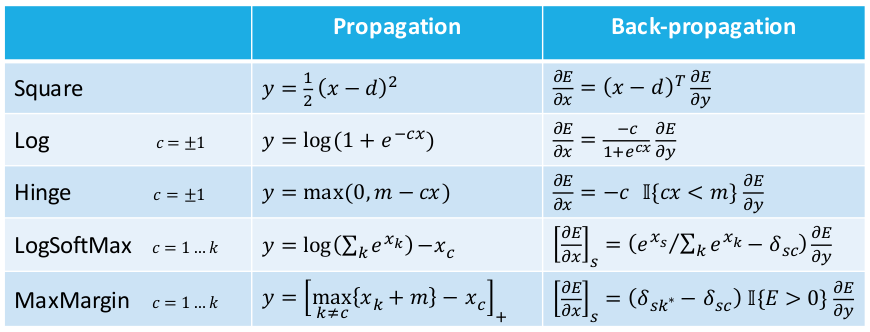Update: As pointed out by commenters, the min and max functions in the ramp formula should be switched.

## 4. Weight initialisation strategy

The current recommended strategy for initialising weights in a neural network is to sample values $$W_{i,j}^{(k)}$$ uniformly from $$[-b,b]$$, where

$$b = \sqrt{\frac{6}{H_k + H_{k+1}}}$$

$$H_k$$ and $$H_{k+1}$$ are the sizes of hidden layers before and after the weight matrix.

## 5. Neural net training tricks

A few practical suggestions from Hugo Larochelle:

• Normalise real-valued data. Subtract the mean and divide by standard deviation.
• Decrease the learning rate during training.
• Can update using mini-batches – the gradient is more stable.
• Can use momentum, to get through plateaus.

If you implemented your backprop by hand and it’s not working, then there’s roughly 99% chance that the gradient calculation has a bug. Use gradient checking to identify the issue. The idea is to use the definition of a gradient: how much will the model error change, if we increase a specific weight by a small amount.

$$\frac{\partial f(x)}{\partial x} \approx \frac{f(x+\epsilon) – f(x-\epsilon)}{2\epsilon}$$

A more in-depth explanation is available here: Gradient checking and advanced optimization

## 7. Motion tracking

Human motion tracking can be done with impressive accuracy. Below are examples from the paper Dynamical Binary Latent Variable Models for 3D Human Pose Tracking by Graham Taylor et al. (2010). The method uses conditional restricted Boltzmann machines.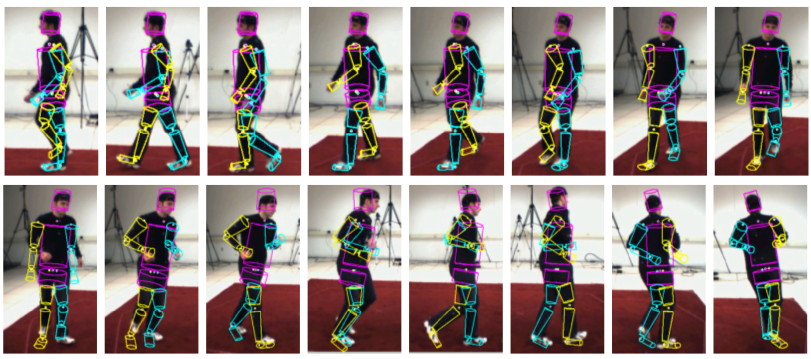## 8. Syntax or no syntax? (aka, “is syntax a thing?”)

Chris Manning and Richard Socher have put a lot of effort into developing compositional models that combine neural embeddings with more traditional parsing approaches. This culminated with a Recursive Neural Tensor Network (Socher et al., 2013), which uses both additive and multiplicative interactions to combine word meanings along a parse tree.

And then, the model was beaten (by quite a margin) by the Paragraph Vector (Le & Mikolov, 2014), which knows absolutely nothing about the sentence structure or syntax. Chris Manning referred to this result as “a defeat for creating ‘good’ compositional vectors”.

However, more recent work using parse trees has again surpassed this result. Irsoy & Cardie (NIPS, 2014) managed to beat paragraph vectors by going “deep” with their networks in multiple dimensions. Finally, Tai et al. (ACL, 2015) have improved the results again by combining LSTMs with parse trees.

The accuracies of these models on the Stanford 5-class sentiment dataset are as follows:

[table width=”500″ colwidth=”100|50″ colalign=”left|center”]
Method, Accuracy
RNTN (Socher et al. 2013), 45.7
Paragraph Vector (Le & Mikolov 2014), 48.7
DRNN (Irsoy & Cardie 2014), 49.8
Tree LSTM (Tai et al. 2015), 50.9
[/table]

So it seems that, at the moment, models using the parse tree are beating simpler approaches. I’m curious to see if and when the next syntax-free approach will emerge that will advance this race. After all, the goal of many neural models is not to discard the underlying grammar, but to implicitly capture it in the same network.

## 9. Distributed vs distributional

Chris Manning himself cleared up the confusion between the two words.

Distributed: A concept is represented as continuous activation levels in a number of elements. Like a dense word embedding, as opposed to 1-hot vectors.

Distributional: Meaning is represented by contexts of use. Word2vec is distributional, but so are count-based word vectors, as we use the contexts of the word to model the meaning.

## 10. The state of dependency parsing

Comparison of dependency parsers on the Penn Treebank:

[table colalign=”left|center|center”]
Parser, Unlabelled Accuracy, Labelled Acccuracy, Speed (sent/s)
MaltParser, 89.8, 87.2, 469
MSTParser, 91.4, 88.1, 10
TurboParser, 92.3, 89.6, 8
Stanford Neural Dependency Parser, 92.0, 89.7, 654
[/table]

The last result is from Google “pulling out all the stops”, by putting massive amounts of resources into training the Stanford neural parser.

## 11. Theano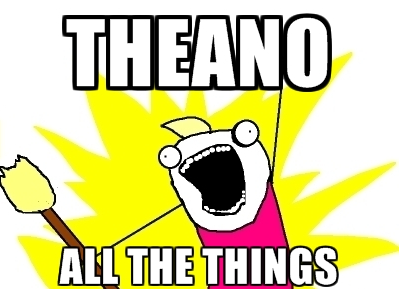Well, I knew a bit about Theano before, but I learned a whole lot more during the summer school. And it is pretty awesome.

Since Theano originates from Montreal, it was especially helpful to be able to ask questions directly from the people who are developing it.

Most of the information that was presented is available online, in the form of interactive python tutorials.

## 12. Nvidia Digits

Nvidia has a toolkit called Digits that trains and visualises complex neural network models without needing to write any code. And they’re selling DevBox – a machine customised for running Digits and other deep learning software (Theano, Caffe, etc). It comes with 4 Titan X GPUs and currently costs \$15,000.

## 13. Fuel

Fuel is a toolkit that manages iteration over your datasets – it can split them into minibatches, manage shuffling, apply various preprocessing steps, etc. There are prebuilt functions for some established datasets, such as MNIST, CIFAR-10, and Google’s 1B Word corpus. It is mainly designed for use with Blocks, a toolkit that simplifies network construction with Theano.

## 14. Multimodal linguistic regularities

Remember “king – man + woman = queen”? Turns out that works with images as well (Kiros et al., 2015).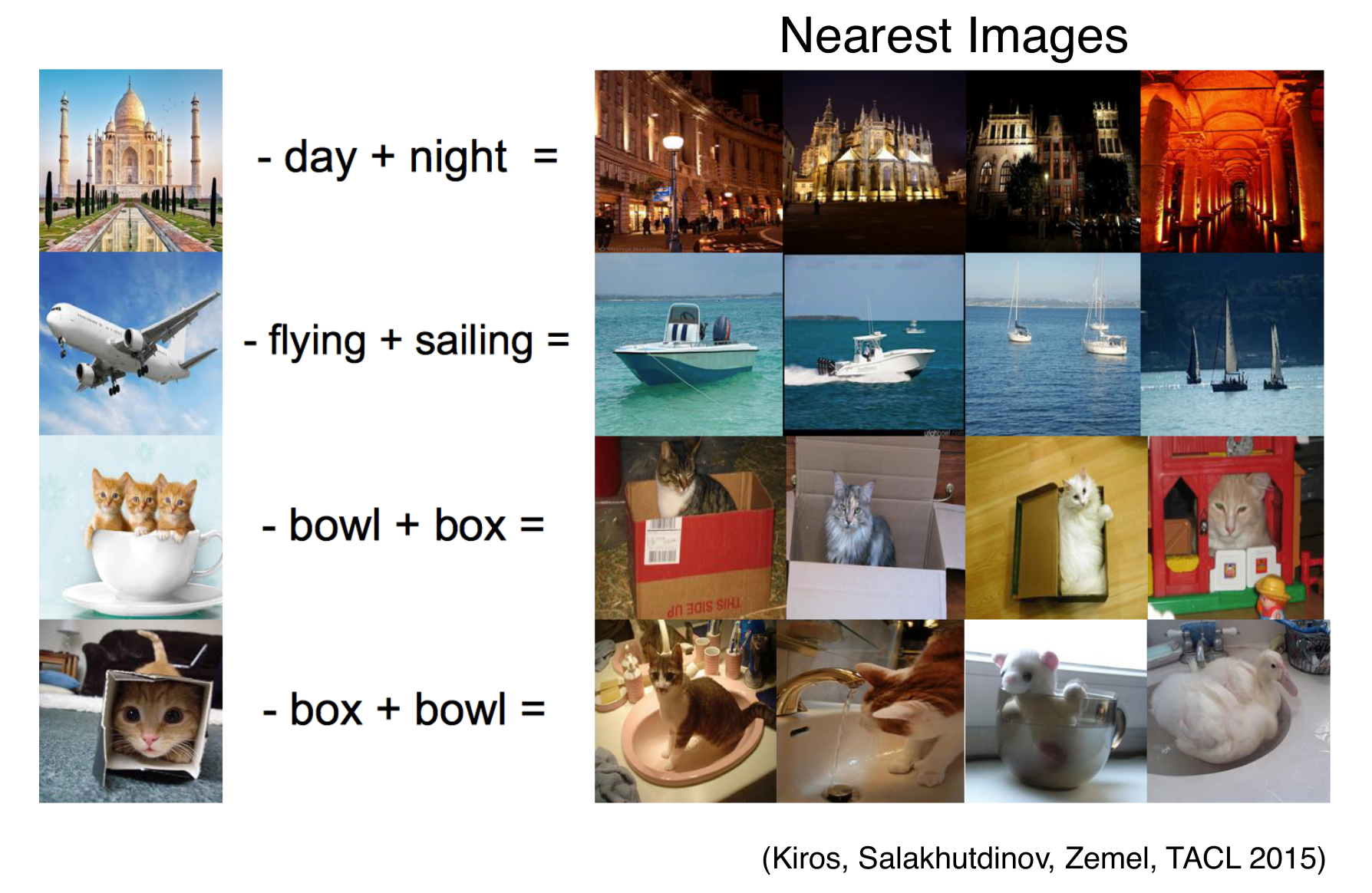## 15. Taylor series approximation

When we are at point $$x_0$$ and take a step to $$x$$, then we can estimate the function value in the new location by knowing the derivatives, using the Taylor series approximation.

$$f(x) = f(x_0) + (x – x_0)f'(x) + \frac{1}{2} (x – x_0)^2 f”(x) + …$$

Similarly, we can estimate the loss of a function, when we update parameters $$\theta_0$$ to $$\theta$$.

$$J(\theta) =J(\theta_0) + (\theta – \theta_0)^T g + \frac{1}{2} (\theta – \theta_0)^T H(\theta – \theta_0) + …$$

where $$g$$ contains the derivatives with respect to $$\theta$$, and $$H$$ is the Hessian with second order derivatives with respect to $$\theta$$.

This is the second-order Taylor approximation, but we could increase the accuracy by adding even higher-order derivatives.

## 16. Computational intensity

Adam Coates presented a strategy for analysing the speed of matrix operations on a GPU. It’s a simplified model that says your time is spent on either reading/writing to memory or doing calculations. It assumes you can do both in parallel so we are interested in which one of them takes more time.

Let’s say we are multiplying a matrix with a vector: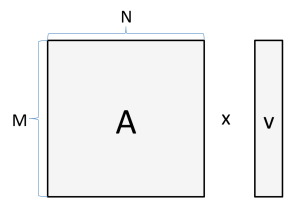If $$M=1024$$ and $$N=512$$, then the number of bytes we need to read and store is:

$$4\text{ bytes }\times (1024 \times 512 + 512 + 1024) = 2.1e6\text{ bytes}$$

And the number of calculations we need to do is:

$$2\times 1024\times 512 = 1e6\text{ FLOPs}$$

If we have a GPU that can do 6 TFLOP/s and has memory bandwidth of  300GB/s, then the total running time will be:

$$\text{max}\{2.1e6\text{ bytes }/ (300e9\text{ bytes}/s), 1e6\text{ FLOPs} / (6e12\text{ FLOP}/s) \} \\ = \text{max}\{ 7\mu s, 0.16\mu s \}$$

This means the process is bounded by the $$7\mu s$$ spent on copying to/from the memory, and getting a faster GPU would not make any difference. As you can probably guess, this situation gets better with bigger matrices/vectors, and when doing matrix-matrix operations.

Adam also described the idea of calculating the intensity of an operation:

Intensity = (# arithmetic ops) / (# bytes to load or store)

In the previous scenario, this would be

Intensity = (1E6 FLOPs) / (2.1E6 bytes) = 0.5 FLOPs/bytes

Low intensity means the system is bottlenecked on memory, and high intensity means it’s bottlenecked by the GPU speed. This can be visualised, in order to find which of the two needs to improve in order to speed up the whole system, and where the sweet spot lies.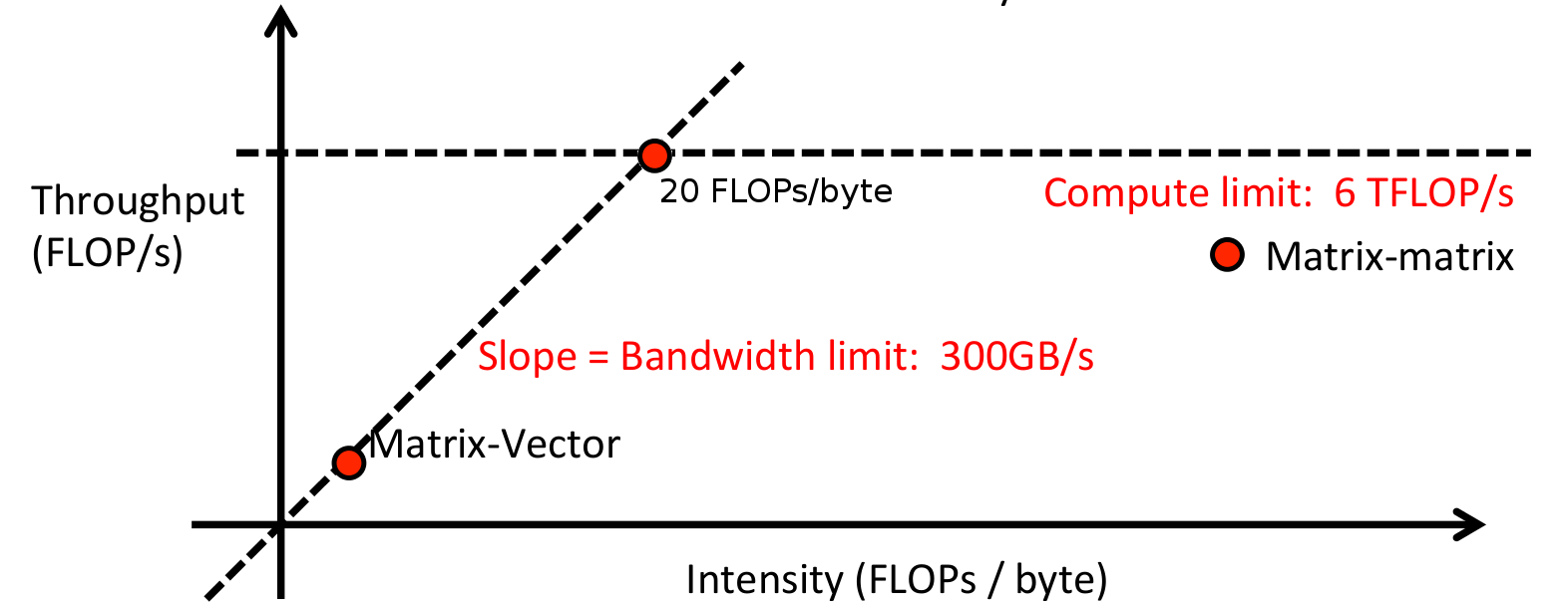## 17. Minibatches

Continuing from the intensity calculations, one way of increasing the intensity of your network (in order to be limited by computation instead of memory), is to process data in minibatches. This avoids some memory operations, and GPUs are great at processing large matrices in parallel.

However, increasing the batch size too much will probably start to hurting the training algorithm and converging can take longer. It’s important to find a good balance in order to get the best results in the least amount of time.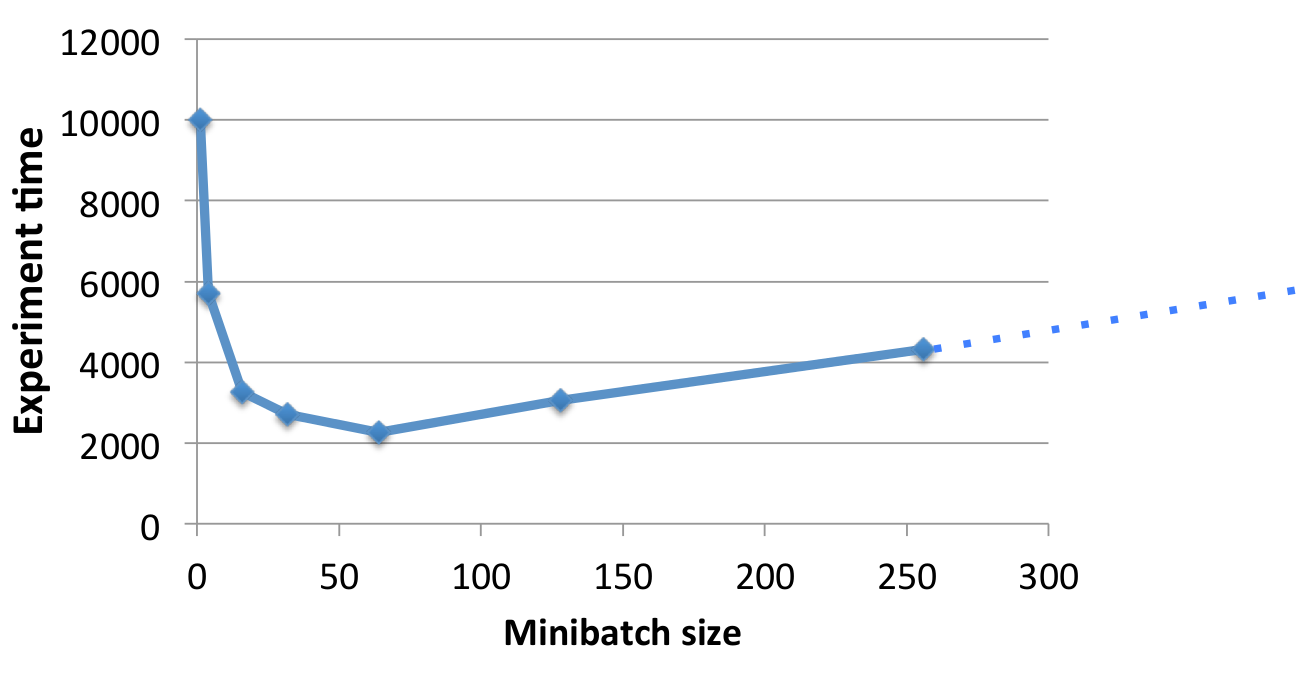## 18. Training on adversarial examples

It was recently revealed that neural networks are easily tricked by adversarial examples. In the example below, the image on the left is correctly classified as a goldfish. However, if we apply the noise pattern shown in the middle, resulting in the image on the right, the classifier becomes convinced this is a picture of a daisy. The image is from Andrej Karpathy’s blog post “Breaking Linear Classifiers on ImageNet”, and you can read more about it there.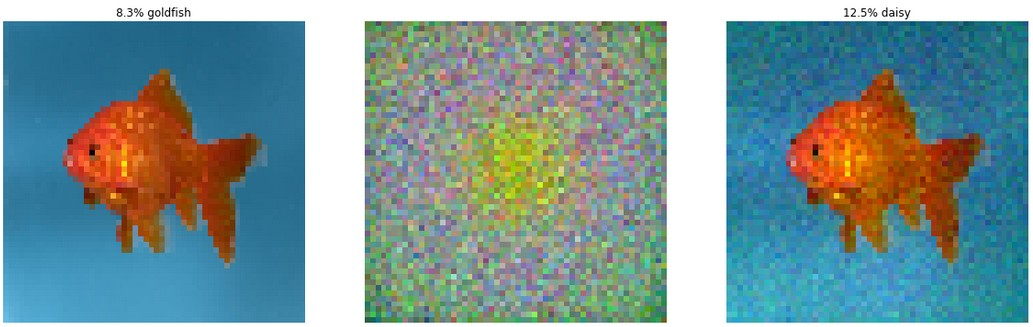The noise pattern isn’t random though – the noise is carefully calculated, in order to trick the network. But the point remains: the image on the right is clearly still a goldfish and not a daisy.

Apparently strategies like ensemble models, voting after multiple saccades, and unsupervised pretraining have all failed against this vulnerability. Applying heavy regularisation helps, but not before ruining the accuracy on the clean data.

Ian Goodfellow presented the idea of training on these adversarial examples. They can be automatically generated and added to the training set. The results below show that in addition to helping with the adversarial cases, this also improves accuracy on the clean examples.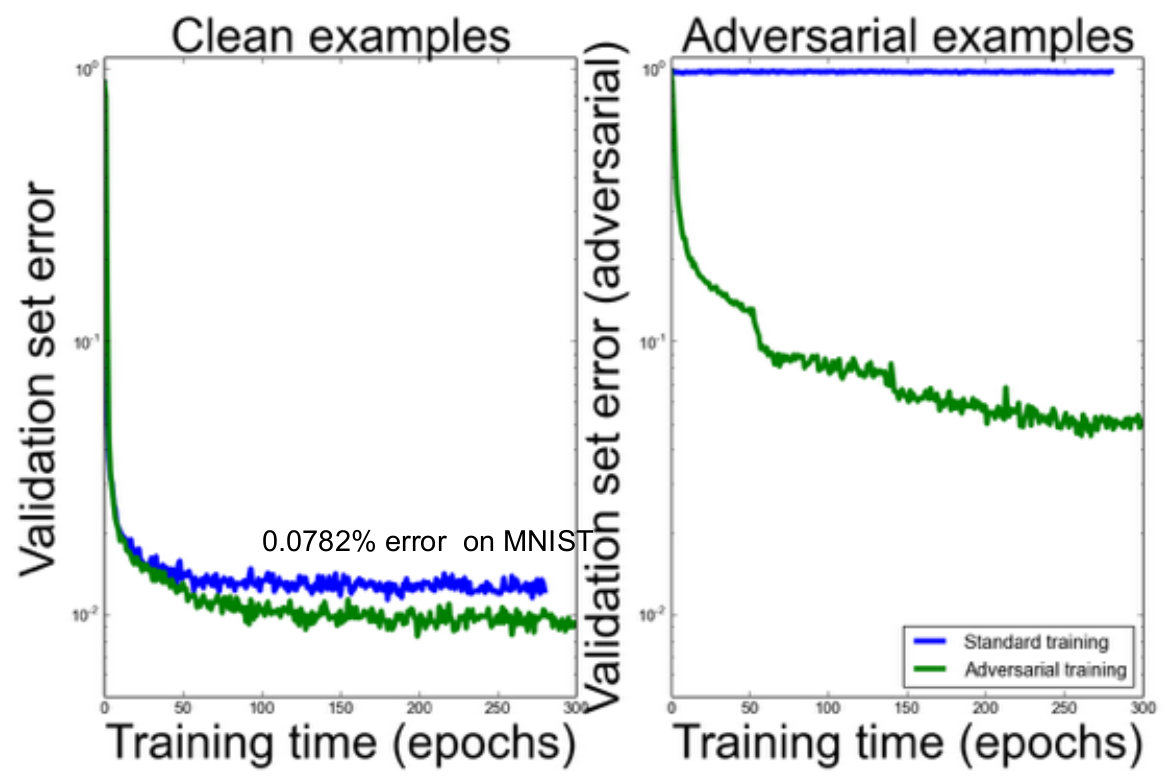Finally, we can improve this further by penalising the KL-divergence between the original predicted distribution and the predicted distribution on the adversarial example. This optimises the network to be more robust, and to predict similar class distributions for similar (adversarial) images.

## 19. Everything is language modelling

Phil Blunsom presented the idea that almost all NLP can be structured as a language model. We can do this by concatenating the output to the input and trying to predict the probability of the whole sequence.

Translation:

$$P(\text{Les chiens aiment les os || Dogs love bones})$$

$$P(\text{What do dogs love? || bones .})$$

Dialogue:

$$P(\text{How are you? || Fine thanks. And you?})$$

The latter two need to be additionally conditioned on some world knowledge. The second part doesn’t even need to be words, but could be labels or some structured output like dependency relations.

## 20. SMT had a rough start

When Frederick Jelinek and his team at IBM submitted one of the first papers on statistical machine translation to COLING in 1988, they got the following anonymous review: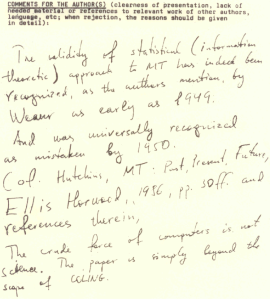The validity of a statistical (information theoretic) approach to MT has indeed been recognized, as the authors mention, by Weaver as early as 1949. And was universally recognized as mistaken by 1950 (cf. Hutchins, MT – Past, Present, Future, Ellis Horwood, 1986, p. 30ff and references therein). The crude force of computers is not science. The paper is simply beyond the scope of COLING.

## 21. The state of Neural Machine Translation

Apparently a very simple neural model can produce surprisingly good results. An example of translating from Chinese to English, from Phil Blunsom’s slides: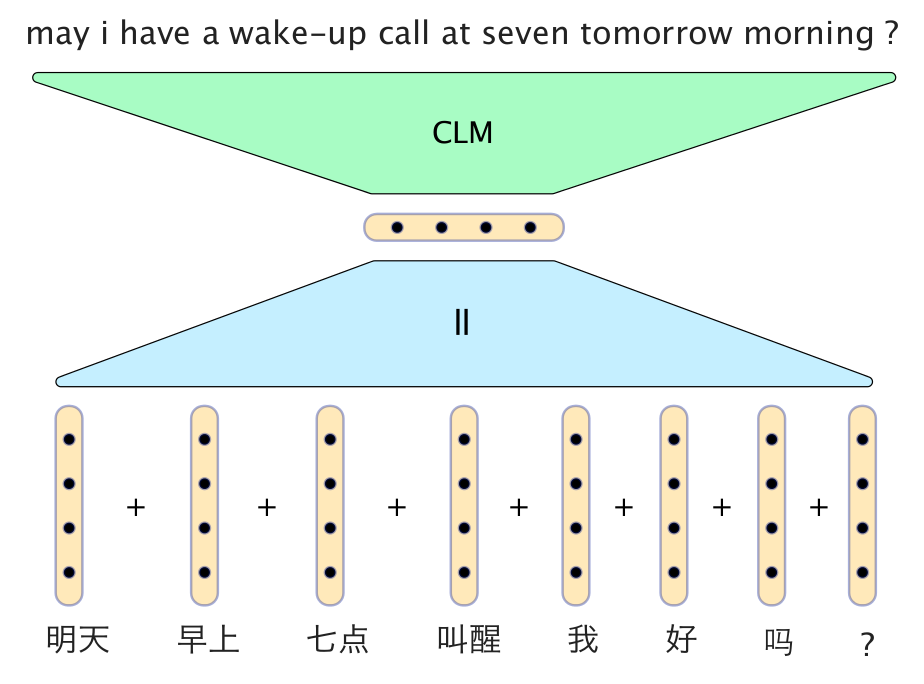In this model, the vectors for the Chinese words are simply added together to form a sentence vector. The decoder consists of a conditional language model which takes the sentence vector, together with vectors from the two recently generated English words, and generates the next word in the translation.

However, neural models are still not outperforming the very best traditional MT systems. They do come very close though. Results from “Sequence to Sequence Learning with Neural Networks” by Sutskever et al. (2014):

[table]
Model, BLEU score
Baseline, 33.30
Best WMT’14 result, 37.0
Scoring with 5 LSTMs, 36.5
Oracle (upper bound), ∼45
[/table]

Update: @stanfordnlp pointed out that there are some recent results where the neural model does indeed outperform the state-of-the-art traditional MT system. Check out “Effective Approaches to Attention-based Neural Machine Translation” (Luong et. al., 2015).

## 22. MetaMind classifier demo

Richard Socher demonstrated the MetaMind image classification demo, which you can train yourself by uploading images. I trained a classifier to detect Edison and Einstein (couldn’t find enough unique images of Tesla). 5 example images for both classes, testing on one held out image each. Seemed to work pretty well.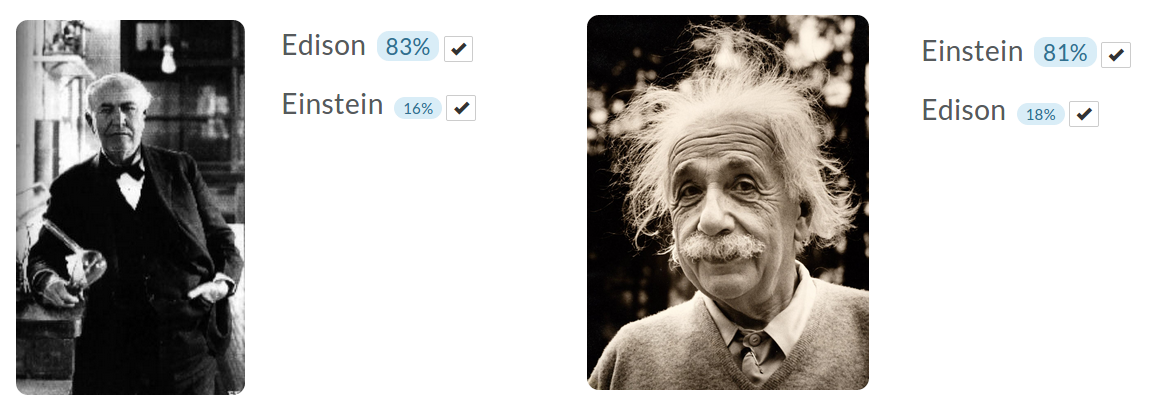Mark Schmidt gave two presentations about numerical optimisation in different scenarios.

In a deterministic gradient method we calculate the gradient over the whole dataset and then apply the update. The iteration cost is linear with the dataset size.

In stochastic gradient methods we calculate the gradient on one datapoint and then apply the update. The iteration cost is independent of the dataset size.

Each iteration of the stochastic gradient descent is much faster, but it usually takes many more iterations to train the network, as this graph illustrates: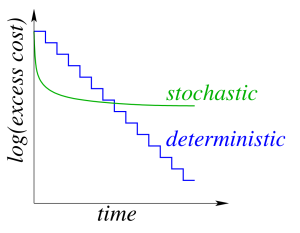In order to get the best of both worlds, we can use batching. More specifically, we could do one pass of the dataset with stochastic gradient descent, in order to quickly get on the right track, and then start increasing the batch size. The gradient error decreases as the batch size increases, although eventually the iteration cost will become dependent on the dataset size again.

Stochastic Average Gradient (SAG) is a method that gets around this, providing a linear convergence rate with only 1 gradient per iteration. Unfortunately, it is not feasible for large neural networks, as it needs to remember the gradient updates for every datapoint, leading to large memory requirements. Stochastic Variance-Reduced Gradient (SVRG) is another method that reduces this memory cost, and only needs 2 gradient calculations per iteration (plus occasional full passes).

Mark said a student of his implemented a variety of optimisation methods (AdaGrad, momentum, SAG, etc). When asked, what he would use in a black box neural network system, the student said two methods: Streaming SVRG (Frostig et al., 2015), and a method they haven’t published yet.

## 24. Theano profiling

If you put “profile=True” into THEANO_FLAGS, it will analyse your program, showing a breakdown of how much is spent on each operation. Very handy for finding bottlenecks.

Following on from Ian Goodfellow’s talk on adversarial examples, Yoshua Bengio talked about having two systems competing with each other.

System D is a discriminative system that aims to classify between real data and artificially generated data.

System G is a generative system, that tries to generate artificial data, which D would incorrectly classify as real.

As we train one, the other needs to get better as well. In practice this does work, although the step needs to be quite small to make sure D can keep up with G. Below are some examples from “Deep Generative Image Models using a Laplacian Pyramid of Adversarial Networks” – a more advanced version of this model which tries to generate images of churches.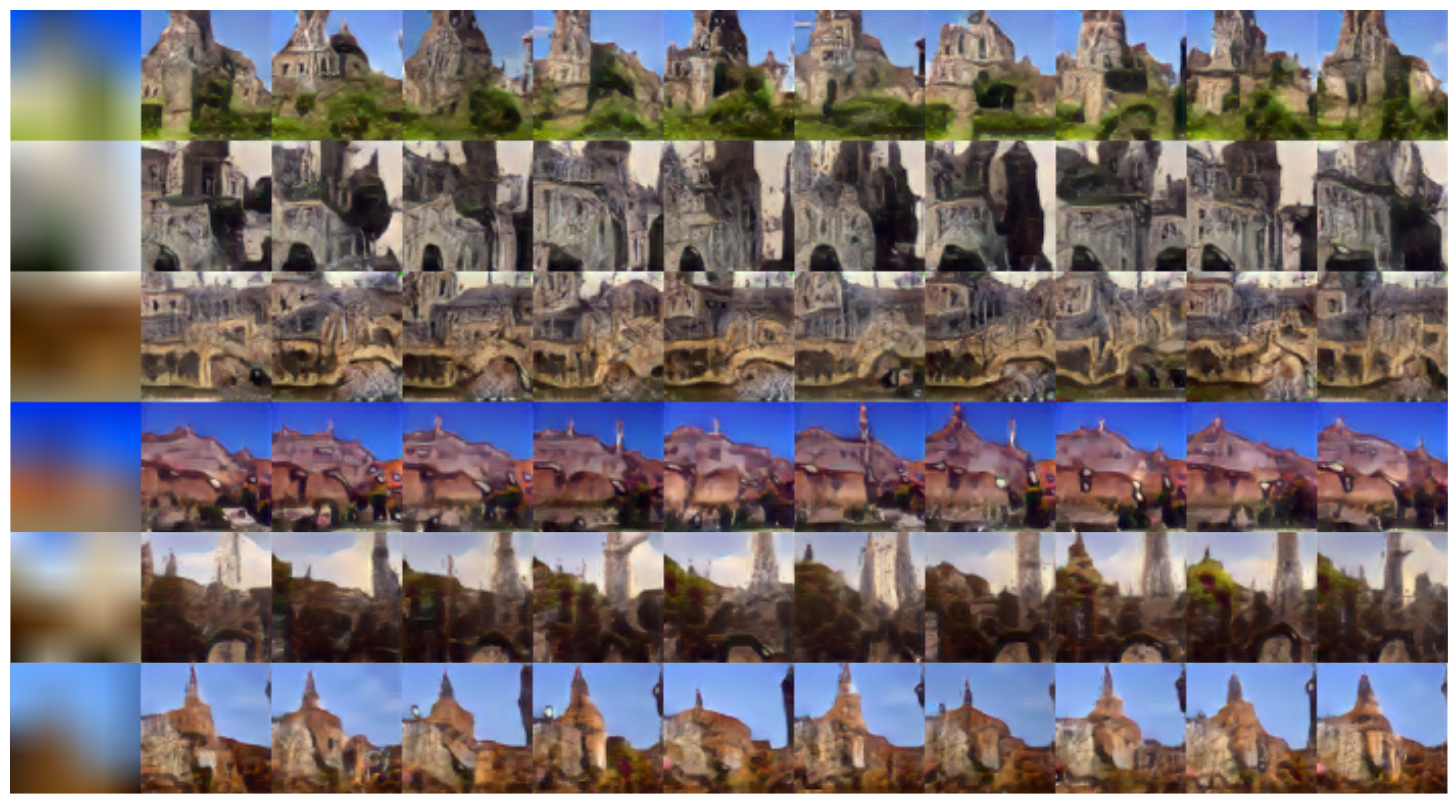## 26. arXiv.org numbering

The arXiv number contains the year and month of the submission, followed by the sequence number. So paper 1508.03854 was number 3854 in August 2015. Good to know.

1.Harri

It seems like min and max are the wrong way around in the definition of `ramp’. Enjoyed the post.

2.Drew Cummins

Nice article! Min and max are backwards in the ramp function, but I guess it’s from someone else’s presentation?

3.Lajanugen

Nice write-up. Found a lot of useful information here.

4.Simon

Excellent post. In the ramp function in 3. I think you have the max and min the wrong way around. The function you have will always output -1.

5.Anh

Hi Marek,

This is a super useful post!! Thanks for sharing cool things @ DL summer school. 🙂

6.Claude Coulombe

Greetings Marek, quite a good summary! I was there and I’ve learned a lot too.

There were also very good talks on NLP and DL by researchers at Google (Phil Blunsom, Ian Goodfellow), Baidu (Adam Cotes), Twitter (Hugo Larochelle), Microsoft (Leon Bottou), MetaMind (Richard Socher) and Stanford University (Chris Manning).

We can add, a lot of material about the different neural network architectures: Autoencoders, RBM (Restricted Boltzmann Machines), CNN (Convolutional Neural Networks), DBM (Deep Boltzmann Machines), DBN (Deep Belief Networks), RNN (Recurrent Neural Networks), LSTM (Long Short Term Memory), etc.

7.petrux

Nice post! Thanks for sharing your experience of the Summer School. If you don’t mind I would like to add a consideration and a question.

So it seems that, at the moment, models using the parse tree are beating simpler approaches. I’m curious to see if and when the next syntax-free approach will emerge that will advance this race. After all, the goal of many neural models is not to discard the underlying grammar, but to implicitly capture it in the same network.

Well, the syntax-free approach needs a larger amount of training data but the the feature engineering effort should be reduced. At the end of the day, it’s just sampling: syntax-free sampling needs more sampling activity, but it’s simpler. And you are right: it will be really interesting to see how this confrontation will evolve.

My question is about the Google dependency parsing. Do you have any link to a deeper description of the project? Thanks a lot!

•Marek

Thanks for the comment.

As far as I know, the details of the Google parsing result have not been released. It was implied at the talk that it was a version of the Stanford parser, modified to take advantage of Google computing capabilities (a bigger model, trained for longer).

•petrux

I see. Thanks for the reply and god luck!

8.Thank you for such great post and lots of great references. I enjoyed the section on neural machine translation the most.

9.Alison Lowndes

Excellent post & Thanks for #12. LOL @ #26 I was like “ohhhh yeahhhh” when I figured that one out too (only about 6 months ago!)

10.Yash

Thanks for sharing these. Couldn’t be there but looks like I can catch up with the online slides and videos.

11.Nitin kandpal

Great post enjoyed It.
Thanks

12.Tony Ma

Are there any videos about the theano tutorials in this summer school?
Thanks!

13.Julien Bélanger

As for 2.Local minima are not a problem in high dimensions can you point me out to the course or paper where this is coming from? Thanks!

•Nikita

you can have a look at this paper and the references therein:
https://arxiv.org/abs/1704.08045

14.Euler

Wow, such a good summary, thank you!

I have a question, why does language model(No. 19) is represented as likelihood?? not a posterior probability.

Thank you again.

•Marek

Actually this is neither a likelihood nor a posterior, but concatenation (||). As the description says, the idea is to predict the probability of the whole sequence (input+output) using a specialised language model.

15.16.Phani

Can Python be used for deep learning since it is used a lot for data science projects in general?

•Marek

Definitely, python is great for deep learning

17.malaysiaexcelr

awesome blog with valuable information

thank you for such an good information.

18.Ricard Luke

Great one

19.repjjnbm

female viagra funny https://menviagraget.com/ viagra cutting pills in half viagra y omega 3 para que es sildenafil 100mg

20.nannie

Hi everyone , I’m Nannie Morris.
Welcome to my website . I started writing in my early school years after a creative writing assignment for my English teacher. I did creative writing for several months before I thought about doing something else.
I had always loved doing non-fiction writing because I’m passionate about learning. When you combine writing talent with a love of learning, research paper writing only makes sense as a job.
I’m passionate about helping the students of the future in their school career. When they get too busy, I am there to help.

Nannie Morris – Academic Writing Help – elearninag.com Corp

21.Tomaser

A customer may know the requirement, but the challenge they face is in reviewing these https://www.the-essays.com/professional-writers companies. In this process, the user may miss common and minute things.

22. Pingback:深度学习Deep Learning 资料大全 – 源码巴士

23. Pingback:【深度学习Deep Learning】资料大全 – 源码巴士